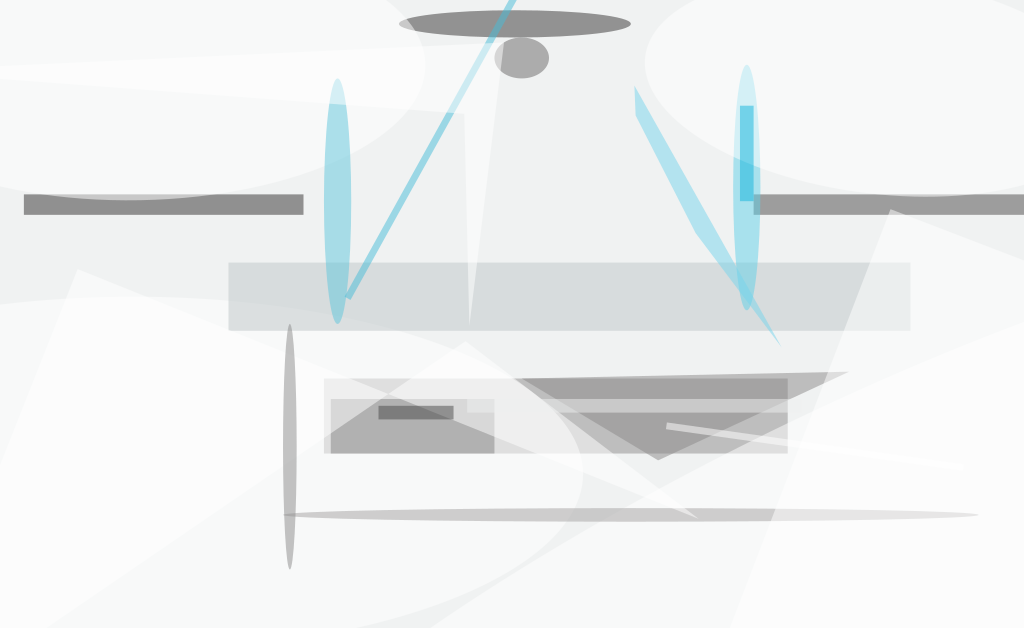According to a proposal, membranes to be used in inflatable focusing mirrors (see Figure 1) would be designed and fabricated with parabolic radial variations of thickness. More specifically, for a mirror membrane with a diameter D, the thickness (t) at a given radial distance (r) from the optical axis would be given by

t = t0(1 + Au2),

where t0 is the thickness at the center, A is a parameter described below, and u ≡ 2r/D. The reason for this proposal is that by suitable choice of A, one could ensure that upon inflation, the membrane would assume a shape that closely approximates a paraboloid — the shape required for focusing in many applications.Figure 1. Two Membranes would be joined at an outer circular edge of diameter D, and the space between them would be pressurized. The upper membrane would be transparent and would serve as a window. The lower membrane would be coated with a reflective material and would serve as a focusing mirror.

Past investigations of membrane mirrors have led to the conclusion that they are not suitable for imaging in visible light because their shapes when inflated differ too much from paraboloids. In particular, upon inflation, a previously flat membrane of uniform thickness assumes an oblate spheroidal shape described by a function named the "Hencky curve" after its discoverer. Although there have been suggestions that radial variations in thickness might result in inflated shapes that equal or closely approximate paraboloids, the necessary variation in thickness has not been published until now.

The proposal is justified by a mathematical derivation that starts with the classical equation for the axial deflection [z(u)] of an initially flat, elastic, thin plate (that is, membrane) of thickness t(u) that is subjected to a differential pressure and restrained by a ring as described above. The problem is to find t(u) such that z(u) would approximate the desired paraboloidal form to the desired degree of precision. The solution involves a numerical integration that leads to the conclusion that a parabolic radial variation of thickness with A = 0.42 would yield the desired inflated shape, regardless of the values of inflation pressure, deflection at the center, and focal ratio of the inflated membrane mirror.

A membrane according to the proposal could be fabricated most conveniently by either of two techniques. One way would be to cast or otherwise form a membrane polymer on a convex spherical mold with a large radius of curvature that yields an acceptably close approximation to the desired shape. The other way would be to adapt a technique that has been used before to make astronomical mirrors: This technique is based on the fact that the height of a liquid on a horizontal, flat, steadily rotating table varies parabolically with radius from the axis of rotation. The table would be covered with a flat disk of glass or other smooth material, a dike to contain liquid would be placed around the periphery of the disk, the required amount of mono-meric liquid would be poured onto the disk, and the disk would be set into rotation at the speed necessary to make the thickness of the liquid at the planned outer diameter of the membrane equal to 1.42 times the thickness at the axis of rotation (see Figure 2). The mon-omer would be polymerized as the table continued to rotate, thereby locking in the parabolic radial variation with thickness.

This work was done by Aden Meinel and Marjorie Meinel of Caltech for NASA's Jet Propulsion Laboratory. For further information, access the Technical Support Package (TSP) free on-line at www.nasatech.com/tsp  under the Mechanics category.

NPO-20952

##### This Brief includes a Technical Support Package (TSP).